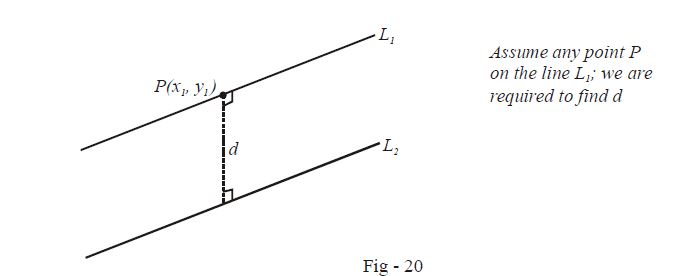# Examples on Length of Perpendicular from a Point to a Line

Go back to  'Straight Lines'

Example – 7

Find the distance between two parallel lines $${{L}_{1}}\,\,and\,\,{{L}_{2}}$$  given by $${{L}_{1}}\equiv ax+by+{{c}_{1}}=0$$ and $${{L}_{2}}\equiv ax+by+{{c}_{2}}=0$$

Solution:Since P lies on L1, we have

\begin{align}&\quad\quad\;\; a{{x}_{1}}+b{{y}_{1}}+{{c}_{1}}=0 \\ & \Rightarrow\quad a{{x}_{1}}+b{{y}_{1}}=-{{c}_{1}} \\ & \Rightarrow \quad a{{x}_{1}}+b{{y}_{1}}+{{c}_{2}}={{c}_{2}}-{{c}_{1}}\qquad\qquad...\text{ }\left( 1 \right) \end{align}

By the previous article, the length of the perpendicular dropped from P upon the line Lis

\begin{align}&\; d=\frac{\left| a{{x}_{1}}+b{{y}_{1}}+{{c}_{2}} \right|}{\sqrt{{{a}^{2}}+{{b}^{2}}}} \\ &\quad =\frac{\left| {{c}_{1}}-{{c}_{2}} \right|}{\sqrt{{{a}^{2}}+{{b}^{2}}}}\qquad\qquad\left( By\text{ }\left( 1 \right)\text{ }above \right) \end{align}

This is the required distance between the two lines

Example – 8

If p and p' be the perpendiculars from the origin upon the lines $${{L}_{1}}\equiv x\sec \theta +y\,\text{cosec}\,\theta -a=0$$ and $${{L}_{2}}\equiv x\cos \theta -y\sin \theta -a\cos 2\theta =0,$$ show that $$4{{p}^{2}}+p{{'}^{2}}={{a}^{2}}$$

Solution: The length of the perpendicular dropped from (0, 0) to L1 is by Art - 7

\begin{align}& p=\frac{\left| a \right|}{\sqrt{{{\sec }^{2}}\theta +\text{cose}{{\text{c}}^{2}}\theta }} \\ &\;\; =\left| a \right|\left| \sin \theta \cos \theta \right|\qquad\qquad...\text{ }\left( 1 \right) \end{align}

Similarly, p', the length of the perpendicular from (0, 0) to L2 is

\begin{align} & p'=\frac{\left| a\cos 2\theta \right|}{\sqrt{{{\cos }^{2}}\theta +{{\sin }^{2}}\theta }} \\ &\;\; =\left| a\cos 2\theta \right|\qquad\qquad\qquad...\text{ }\left( 2 \right) \end{align}

We now have from (1) and (2)

\begin{align} & \;4{{p}^{2}}+{{p}^{'2}}=4{{a}^{2}}{{\sin }^{2}}\theta +{{a}^{2}}{{\cos }^{2}}2\theta \\ & \qquad\qquad={{a}^{2}}{{\sin }^{2}}2\theta +{{a}^{2}}{{\cos }^{2}}2\theta \\ &\qquad \qquad={{a}^{2}} \end{align}

Straight Lines
Straight Lines
grade 11 | Questions Set 1
Straight Lines
Straight Lines
grade 11 | Questions Set 2